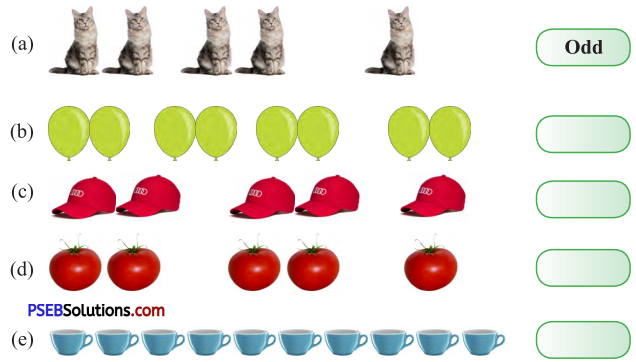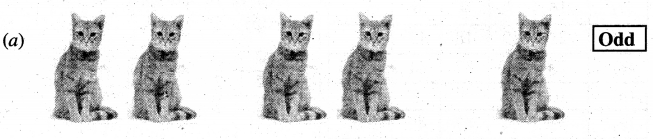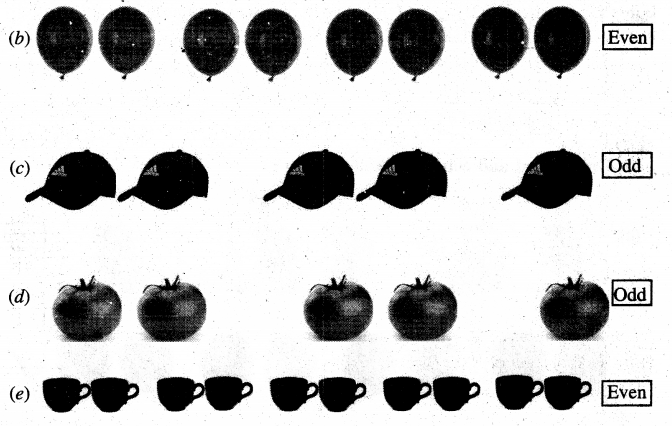# PSEB 5th Class Maths Solutions Chapter 3 HCF and LCM Ex 3.1

Punjab State Board PSEB 5th Class Maths Book Solutions Chapter 3 HCF and LCM Ex 3.1 Textbook Exercise Questions and Answers.

## PSEB Solutions for Class 5 Maths Chapter 3 HCF and LCM Ex 3.1

Question 1.
Write the first five multiples of the following numbers :
(a) 5
(b) 9
(c) 10
(d) 12
(e) 16
(f) 17
Solution:
(a) Multiples of 5 = 5, 10, 15, 20, 25
(b) Multiples of 9 = 9, 18, 27, 36, 45
(c) Multiples of 10 = 10, 20, 30, 40, 50
(d) Multiples of 12 = 12, 24, 36, 48, 60
(e) Multiples of 16 = 16, 32,48, 64, 80
(f) Multiples of 17 = 17, 34, 51, 68, 85

Question 2.
Find the factors of the given numbers :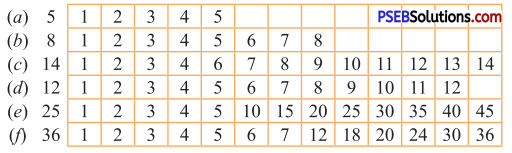Solution:
(a) 1, 5
(b) 1, 2, 4, 8
(c) 1, 2, 7, 14
(d) 1, 2, 3, 4, 6, 12
(e) 1, 5, 25
(f) 1, 2, 3, 4, 6, 9, 12, 18, 36Question 3.
Write the factors of the following numbers :
(a) 18
(b) 24
(c) 35
(d) 36
(e) 45
(f) 21
Solution:
(a) 1, 2, 3, 6, 9, 18
(b) 1, 2, 3, 4, 6, 8, 12, 24
(c) 1, 5, 7, 35
(d) 1, 2, 3, 4, 6, 9, 12, 18, 36
(e) 1, 3, 5, 9, 15, 45
(f) 1, 3, 7, 21.

Question 4.
Find out the even numbers from the following :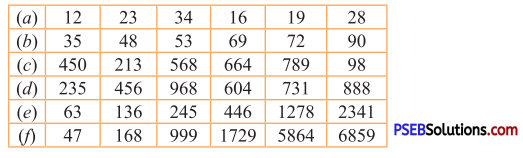Solution:
(a) 12, 34, 16, 28
(b) 48, 72, 90
(c) 450, 568, 664, 98
(d) 456, 968, 604, 888
(e) 136, 446, 1278
(f) 168, 5864.

Question 5.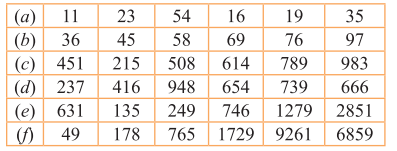Solution:
(a) 11, 23, 19, 35
(b) 45, 69, 97
(c) 451, 215, 789, 983
(d) 237, 739
(e) 631, 135, 249, 1279, 2851
(f) 49, 765, 1729, 9261, 6859.

Question 6.
Fill in the blanks :
(a) If 4 × 9 = 36 then factors of 36 are …….. and ………
(b) If 8 × 7 = 56 then factors of 56 are ……… and ……….
(c) If 3 × 5 × 6 = 90 then factors of 90 are ……., ……. and …….
(d) If 8 × 10 = 80, the multiple of 8 and 10 is ……….
(e) If 2 × 3 × 5 = 30, then 30, is the multiple of …….., ………. and ……..
Solution:
(a) 4, 9
(b) 8, 7
(c) 3, 5, 6
(d) 80
(e) 2, 3, 5.

Question 7.
Write True or False:

(a) 24 is a factor of 24. ____
(b) 2 is a factor of every number. ____
(c) 24 is an even number. ____
(d) 136 is an odd number. ____
(e) There are infinite multiples of a number. ____
(f) 36 is a multiple of 5 and 7. ____
(g) Sum of two even numbers is an odd number. ____
(h) Smallest even number is 0. ____
(i) 152 is an odd number. ____
(j) There are five single digit even numbers. ____
Solution:
(a) True
(b) False
(c) True
(d) False
(e) True
(f) False
(g) False
(h) False
(i) False
(j) FalseQuestion 8.
Observe the Factorisation Pattern and complete :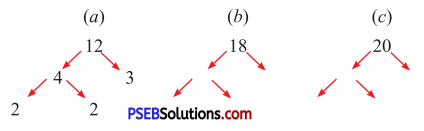Solution: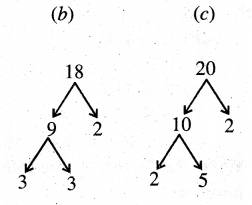Question 9.
Look at the pattern and solve: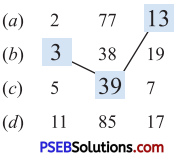Solution: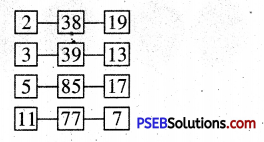Question 10.
Understand and complete the following: